# Search PBS Space Time

## Results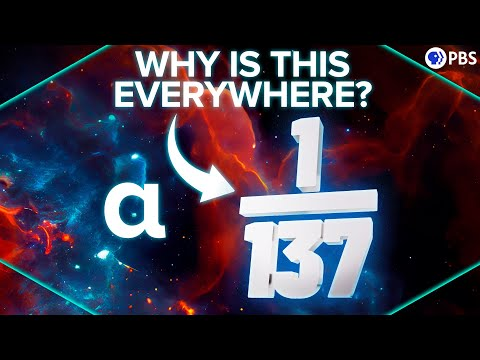### 2022-09-28: Why Is 1/137 One of the Greatest Unsolved Problems In Physics?

• 05:14: ... they can interact in many different ways, which we can visualize with Feynman Diagrams. ...
• 06:02: ... a photon, or in the case of two electrons interacting by,  say Feynman diagrams - it’s the base probability at each vertex, each interaction between ...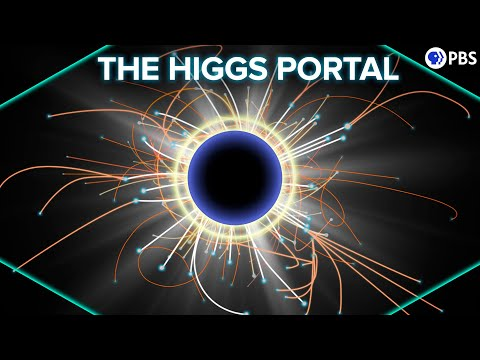### 2022-09-14: Could the Higgs Boson Lead Us to Dark Matter?

• 02:10: We can bundle the different methods into three broad categories, each represented by a different Feynman diagram.
• 02:17: ... Feynman diagram is just a way to represent the interactions of particles, plotting time ...
• 03:35: The second family of experiments, indirect detection, can be represented by rotating our Feynman diagram.
• 04:41: OK, let’s try rotating our Feynman diagram one more time.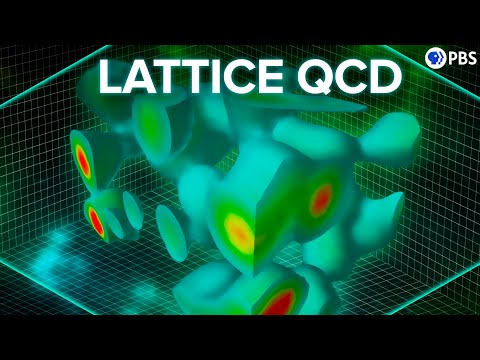### 2022-08-03: What Happens Inside a Proton?

• 03:12: ... Each family of interaction types is represented by a Feynman diagram, and quantum electrodynamics   gives us a recipe book for ...
• 04:17: ... include the simplest few levels.   Each pair of vertices on a Feynman diagram represents the probability of a pair of   electrons interacting with the ...
• 05:31: ... photons of the electromagnetic field.   We can draw Feynman diagrams of these  interactions, now with curly gluon lines.   ...
• 06:02: ... have the luxury of throwing away all but the simplest   Feynman diagrams when you’re calculating the interaction probability for your pair ...
• 06:37: ... In fact it’s extremely challenging to  do QCD with the Feynman diagram approach   even with computers. Now Before any ...
• 07:07: ... quarks, how can we even test the theory?  Well, we need to abandon Feynman diagrams.   In fact, we need to abandon the idea of particles altogether. See, ...
• 07:53: ... the course of a strong force interaction.   Similar to how Feynman diagrams work, to do this you need to account for all possible paths ...
• 08:30: ... could do that even given the entire life of  the universe. For QED, Feynman diagrams let   us reduce the number of field configurations by ...
• 08:58: ... now.   Every time you add the probability  for a single Feynman diagram   you’re actually adding infinite possible  trajectories using ...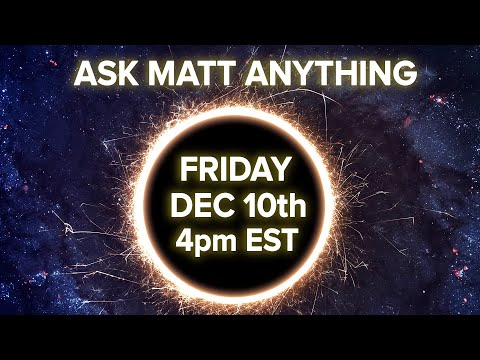### 2021-12-10: 2021 End of Year AMA!

• 00:02: ... between two particles whether they're repelled or attracted using feynman diagrams okay so what you do is you sum over you add over all the possible ways ...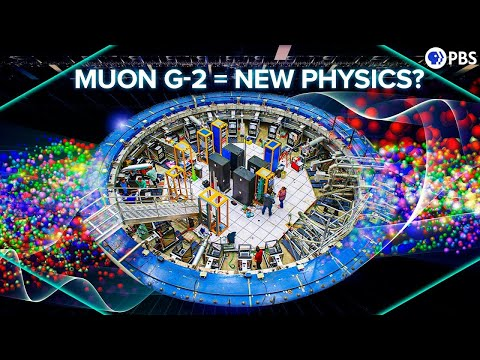### 2021-04-07: Why the Muon g-2 Results Are So Exciting!

• 05:02: We depict these interactions in Feynman diagrams.
• 05:05: Each Feynman diagram represents one family of ways that the interaction could proceed.
• 05:10: And the sum of all possible Feynman diagrams, gives you the interaction strength.
• 05:14: For a deeper dive in Feynman diagrams, virtual particles, and quantum electrodynamics, we have you covered, episode list in the description.
• 05:23: We can represent an electron interacting with a magnetic field, with the simplest possible Feynman diagram.
• 05:29: Really not even a full Feynman diagram.
• 06:12: Each layer of complication involve many more Feynman diagrams, but also added less and less to the interaction.
• 06:19: The latest calculations rely on powerful computers to add many thousands of Feynman diagrams, and get us our g-factor to 12 significant figures.
• 07:36: So you add up all of the Feynman diagram to all possible electromagnetic interactions of the muon, but you don't stop there.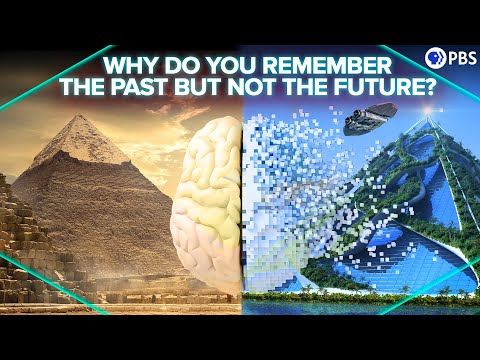### 2020-12-08: Why Do You Remember The Past But Not The Future?

• 04:34: We’re showing this in the style of a Feynman diagram.
• 05:48: We can see that when we flip the Feynman diagram on its head - it's symmetric.
• 06:02: ... “Feynman diagram” of our asteroid looks like countless particles coming together in many ...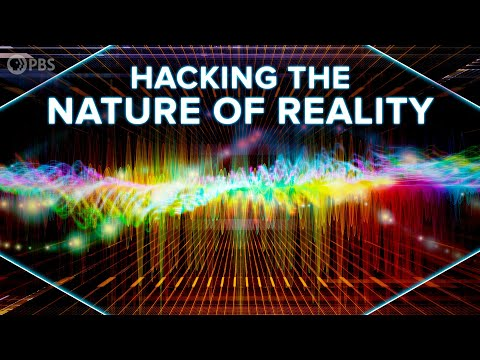### 2020-01-27: Hacking the Nature of Reality

• 07:23: But in order to avoid those sums of Feynman diagrams, S-matrix theory also relies on symmetries between those virtual interactions.
• 07:36: ... matter traveling backwards in time - that folds together large sets of Feynman diagram and helps us ignore the actual causal structure within the interaction ...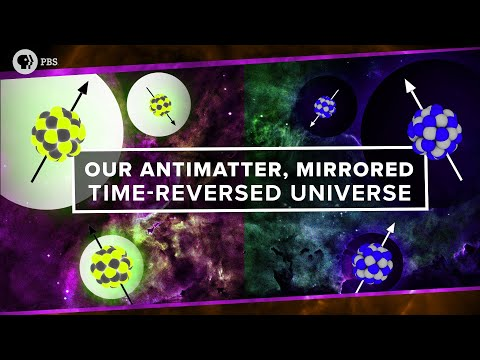### 2019-01-16: Our Antimatter, Mirrored, Time-Reversed Universe

• 10:26: ... it's essential to his path integral approach to quantum mechanics and to Feynman diagrams maybe that's why he was so into building antimatter clocks. So, yeah the ...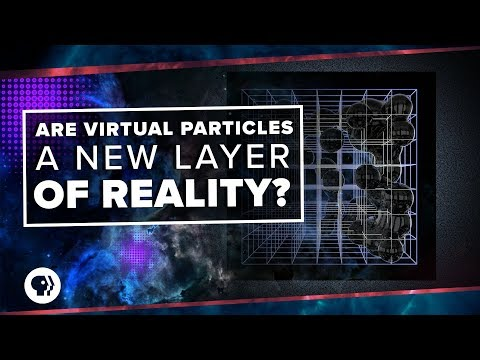### 2018-10-31: Are Virtual Particles A New Layer of Reality?

• 04:33: Maybe you recognize these things-- Feynman diagrams.
• 04:58: ... Feynman diagrams are an absolutely essential tool in most modern quantum field theory ...
• 08:22: In fact, you only see that force in the sum of all possible virtual photons over all possible Feynman diagrams.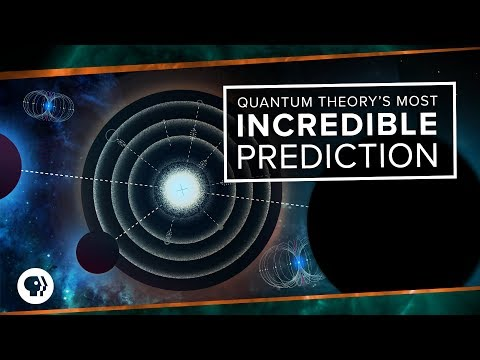### 2018-08-15: Quantum Theory's Most Incredible Prediction

• 08:08: In particular, we've been at Feynman diagrams, which are our best tool for dealing with the absurd complexity of quantum fields.
• 08:30: A basic interaction of an electron with an EM field is illustrated by this partial Feynman diagram.
• 10:18: For each new degree of precision, the number of Feynman diagrams needed explodes.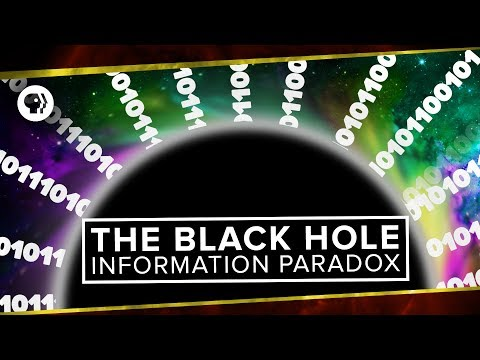### 2018-06-20: The Black Hole Information Paradox

• 14:28: Check out our episode on the path integral and Feynman diagrams for more info on this wackiness.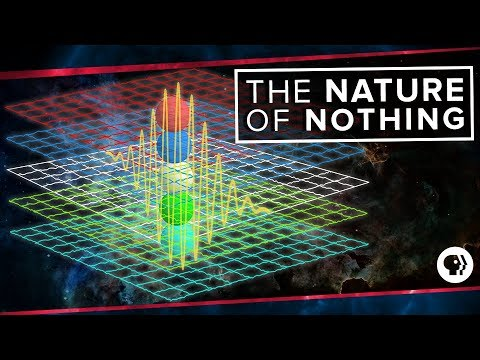### 2017-10-19: The Nature of Nothing

• 04:41: Virtual particles are the links governing all particle interactions in the famous Feynman diagrams.### 2017-08-16: Extraterrestrial Superstorms

• 11:50: Last week, we talked about John Archibald Wheeler's one electron universe idea as well as gave the solution to the Feynman diagram challenge.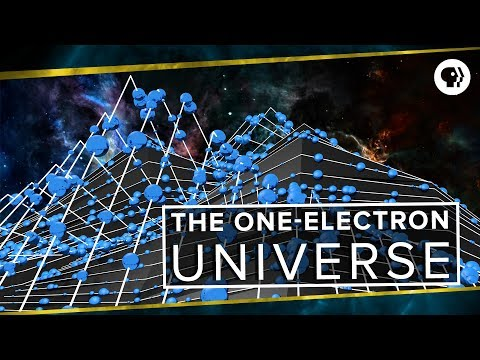### 2017-08-10: The One-Electron Universe

• 06:22: ... field theory calculations, because it massively cuts down the number of Feynman diagrams you ...
• 07:18: ... we draw a Feynman diagram for the whole universe, we can have only one electron undergo countless ...
• 09:13: ... nature of anti-matter, let's go back and look at the solution to the Feynman diagram challenge ...
• 09:22: ... scatter off each other using the simple rules I laid out for building Feynman diagrams. ...### 2017-08-02: Dark Flow

• 09:54: Last week, we talked about the actual rules by which Feynman diagrams can be used to describe real interactions in quantum electrodynamics.
• 10:04: ... couple of you pointed out that the Feynman diagram vertex, representing interactions between an electron, positron, and ...
• 10:20: In fact, vertices are really just building blocks for Feynman diagrams.
• 11:57: ... happens after the electron undergoes an interaction, represented by a Feynman diagram, then we need to think of that retro-causal influence as also propagating ...
• 12:14: Squid Master started studying physics and got a tattoo of a Feynman diagram, then switched majors to economics.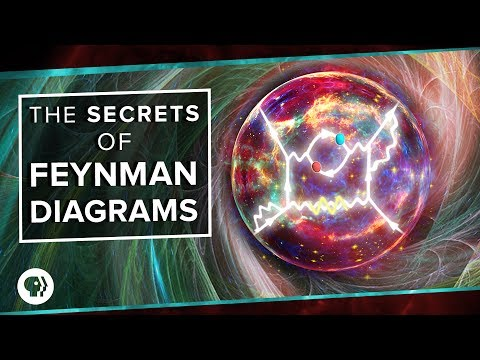### 2017-07-26: The Secrets of Feynman Diagrams

• 00:03: ... Feynman diagrams revolutionized particle physics by providing a simple system to sort out ...
• 01:12: ... as we discussed in our episode on solving impossible equations, the Feynman diagrams allow physicists to quickly figure out which of the infinite ...
• 01:38: The miracle of Feynman diagrams is that an absurdly simple set of rules allows you to easily find all of the important interactions.
• 02:19: In Feynman diagrams, we depict the electron as an arrow pointing forwards in time, while the positron is an arrow pointing backwards in time.
• 02:40: Throw these on a plot of space versus time, and we have a Feynman diagram-- a useless one.
• 03:25: And it can be used to construct infinite Feynman diagrams.
• 05:25: The overall interaction described by a set of Feynman diagrams is defined by the particles going in and the particles going out.
• 07:37: Part of the beauty of Feynman diagrams is that each of these diagrams themselves represents an infinite number of specific interactions.
• 09:43: These may seem like wildly different processes, but in the math represented by Feynman diagrams, they're exactly the same.
• 10:02: ... fact makes Feynman diagrams an incredibly powerful tool in simplifying quantum field theory ...
• 10:27: But for now, I want to give you a chance to play with Feynman diagrams yourselves.
• 10:41: The most important Feynman diagrams for Bhabha scattering are the two cases involving a single virtual photon.
• 11:21: Send your neatly drawn Feynman diagrams to pbsspacetime@gmail.com within one week of the release of this episode.
• 11:29: Include in the subject line the words Feynman diagram challenge, because we filter by subject line.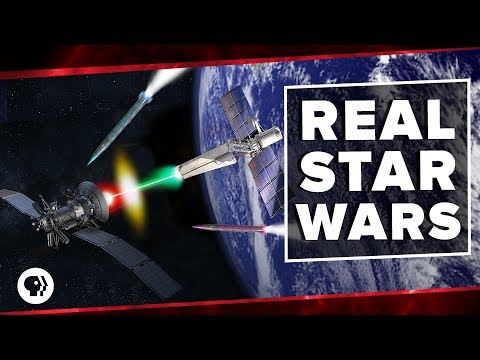### 2017-07-19: The Real Star Wars

• 16:27: When we draw the Feynman diagrams for electron scattering, we need to include separate diagrams in which the ingoing electrons swap places.
• 16:37: A few of you asked why it is that each additional vertex reduces the probability contribution of a given Feynman diagram by around a factor of 100.### 2017-07-12: Solving the Impossible in Quantum Field Theory

• 00:23: The most famous of these are the incredible Feynman diagrams.
• 01:16: Finally, the entire mess was ordered into a system that mere humans could deal with using the famous Feynman diagrams.
• 03:04: This is a good time to introduce our first Feynman diagram.
• 03:18: In a Feynman diagram, one direction is the time-- in this case, up.
• 03:38: But Feynman diagrams aren't really just drawings of the interaction.
• 03:46: Each part of the Feynman diagrams represents a chunk of the math.
• 05:18: And this is where Feynman diagrams start to come in handy, because they keep track of the different families of possibilities.
• 06:46: Here, Feynman diagrams are indispensable.
• 08:00: So with Feynman diagrams, you very quickly get an idea of which are the important additions to your equation and which you can ignore.
• 08:09: Perturbation theory, with the help of Feynman diagrams, make the calculation possible, but that doesn't mean we're done.
• 11:08: ... Feynman diagrams successfully describe everything from particle scattering, self-energy ...
• 11:20: We'll go further into the nuts and bolts of Feynman diagrams in an upcoming challenge episode.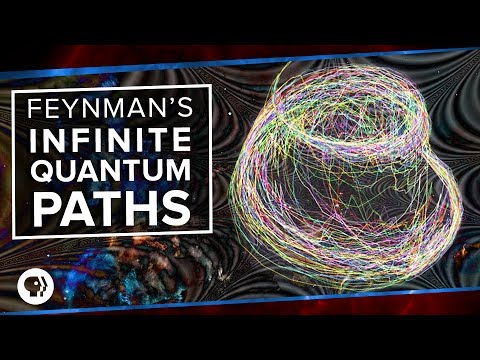### 2017-07-07: Feynman's Infinite Quantum Paths

• 11:12: One powerful tool in making sense of these infinite possible events also came from Richard Feynman, specifically Feynman diagrams.
19 result(s) shown.